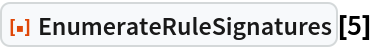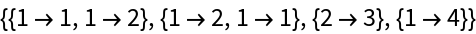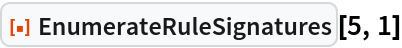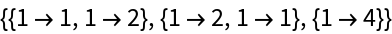#Function Repository Resource:

# EnumerateRuleSignatures

Enumerate possible rule signatures for substitution systems, Wolfram models, etc.

Contributed by: Stephen Wolfram
 ResourceFunction["EnumerateRuleSignatures"][n] gives a list of rule signatures involving an overall total of n elements. ResourceFunction["EnumerateRuleSignatures"][n,s] gives only cases where at least one left-hand side is at most s.

## Examples

### Basic Examples (2)

Enumerate rule signatures with an overall total of 5 elements:

 In:=Out=Include only cases where at least one left-hand side has length no more than 1:

 In:=Out=